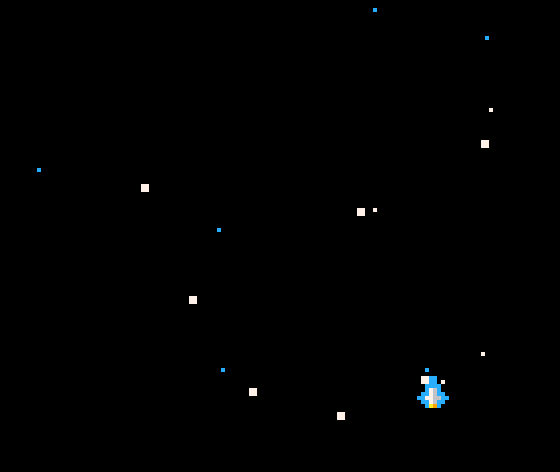# Friction and Inertia in Computer Games: Making things driftOne of the best ways to make a computer game feel ‘real’ is to add inertia to your characters.  In order to do this you have to move away from directly positioning them and instead control their velocity instead.

A crucial aspect to velocity is that it is a vector quantity, that is it represents speed in a particular direction.  To control a character in a 2D game you need to have speed in the x and y directions (for 3D you would need speed in the z direction as well).

At the beginning of the game, I declare a pair of variables for the velocity.

```--velocity in the x and y directions
vx=0
vy=0```

It is also important to implement friction in your game.  In a space game, there is the possibility that you would have no friction (check out Asteroids, for example) but even here, for the sake of playability, it is worth having friction.  Friction is the game is a percentage that represents how much speed is maintained each time it is checked, so a value of 0.5 means that only 50% of the speed is kept each turn (this would be a high level of friction) whereas a value of 0.99 means that only 1% of the speed is lost each turn.

Here is an example of both:So, under the variables for speed with add friction

```--velocity in the x and y directions
vx=0
vy=0

--friction
friction = 0.9```

Now we simply control the position of the character by adding the veocity for each direction to the x and y positions.  Then we decrease each velocity by the friction value.  This code would all go in your game loop (i.e. step in GameMaker or _update() in PICO-8).

```--move the charcter
x+=vx
y+=vy

--decrease the speed
vx*=friction
vy*=friction
```

Finally, to simulate inertia we need to set the velocity slowly, in other words, we need an acceleration that will apply to the object when we press a button.

So, back in the initialization section, we add an acceleration variable.  I assume that acceleration by the craft is the same in every direction, so I am using one variable (accn).

```--velocity in the x and y directions
vx=0
vy=0

--friction
friction = 0.9

--acceleration
accn=0.1```

Then, in the game loop, I respond to a button press by adding the acceleration to the speed

```--apply acceleration
if btn(0) then vx-=accn end
if btn(1) then vx+=accn end
if btn(2) then vy-=accn end
if btn(3) then vy+=accn end

--move the charcter
x+=vx
y+=vy

--decrease the speed
vx*=friction
vy*=friction```

The example above uses PICO-8 syntax, but how you respond to the acceleration is up to you.  Sometimes, to prevent the craft travelling to fast it is worth putting in a maximum velocity in the original settings.

```--velocity in the x and y directions
vx=0
vy=0

--friction
friction = 0.9

--acceleration
accn=0.1

--maximum velocity
max_vel=2```

This needs to be checked, but obviously you could be moving with a negative velocity (as velocity is a vector and contains direction as well as magnitude).  To test whether we exceed the maximum velocity, we need to use the absolute function.  Again, using PICO-8 as an example

```--apply acceleration
if btn(0) and abs(vx)<max_vel then vx-=accn end
if btn(1) and abs(vx)<max_vel then vx+=accn end
if btn(2) and abs(vy)<max_vel then vy-=accn end
if btn(3) and abs(vy)<max_vel then vy+=accn end

--move the charcter
x+=vx
y+=vy

--decrease the speed
vx*=friction
vy*=friction```

Putting these two together gives realistic movement to your games.

Happy programming!